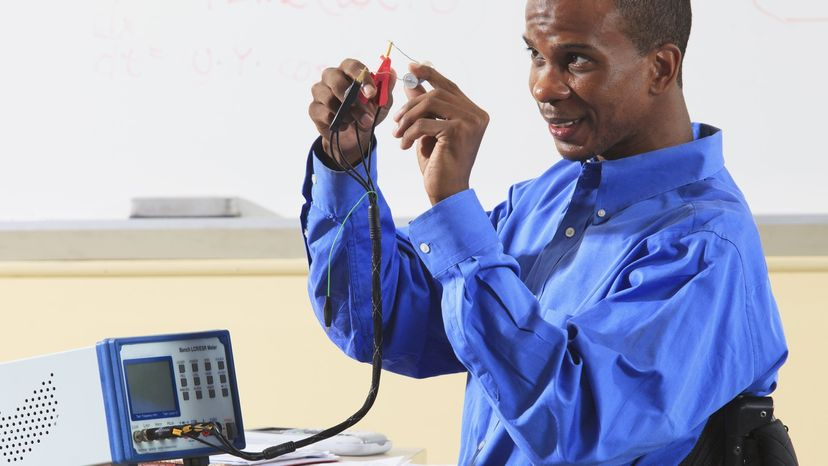# How Inductors WorkOne big use of inductors is to team them up with capacitors to create oscillators. Huntstock / Getty Images

An inductor is about as simple as an electronic component can get -- it is simply a coil of wire. It turns out, however, that a coil of wire can do some very interesting things because of the magnetic properties of a coil.

## Inductor BasicsIn a circuit diagram, an inductor is shown like this:

To understand how an inductor can work in a circuit, this figure is helpful:What you see here is a battery, a light bulb, a coil of wire around a piece of iron (yellow) and a switch. The coil of wire is an inductor. If you have read How Electromagnets Work, you might recognize that the inductor is an electromagnet.

If you were to take the inductor out of this circuit, what you would have is a normal flashlight. You close the switch and the bulb lights up. With the inductor in the circuit as shown, the behavior is completely different.

­The light bulb is a resistor (the resistance creates heat to make the filament in the bulb glow -- see How Light Bulbs Work for details). The wire in the coil has much lower resistance (it's just wire), so what you would expect when you turn on the switch is for the bulb to glow very dimly. Most of the current should follow the low-resistance path through the loop. What happens instead is that when you close the switch, the bulb burns brightly and then gets dimmer. When you open the switch, the bulb burns very brightly and then quickly goes out.

The reason for this strange behavior is the inductor. When current first starts flowing in the coil, the coil wants to build up a magnetic field. While the field is building, the coil inhibits the flow of current. Once the field is built, current can flow normally through the wire. When the switch gets opened, the magnetic field around the coil keeps current flowing in the coil until the field collapses. This current keeps the bulb lit for a period of time even though the switch is open. In other words, an inductor can store energy in its magnetic field, and an inductor tends to resist any change in the amount of current flowing through it.

## Henries

The capacity of an inductor is controlled by four factors:

• The number of coils - More coils means more inductance.
• The material that the coils are wrapped around (the core)
• The cross-sectional area of the coil - More area means more inductance.
• The length of the coil - A short coil means narrower (or overlapping) coils, which means more inductance.

Putting iron in the core of an inductor gives it much more inductance than air or any non-magnetic core would.

The standard unit of inductance is the henry. The equation for calculating the number of henries in an inductor is:

H = (4 * Pi * #Turns * #Turns * coil Area * mu) / (coil Length * 10,000,000)

­ The area and length of the coil are in meters. The term mu is the permeability of the core. Air has a permeability of 1, while steel might have a permeability of 2,000.

## Inductor Application: Traffic Light Sensors

Let's say you take a coil of wire perhaps 6 feet (2 meters) in diameter, containing five or six loops of wire. You cut some grooves in a road and place the coil in the grooves. You attach an inductance meter to the coil and see what the inductance of the coil is.

Now you park a car over the coil and check the inductance again. The inductance will be much larger because of the large steel object positioned in the loop's magnetic field. The car parked over the coil is acting like the core of the inductor, and its presence changes the inductance of the coil. Most traffic light sensors use the loop in this way. The sensor constantly tests the inductance of the loop in the road, and when the inductance rises it knows there is a car waiting!

Usually you use a much smaller coil. One big use of inductors is to team them up with capacitors to create oscillators. See How Oscillators Work for details.